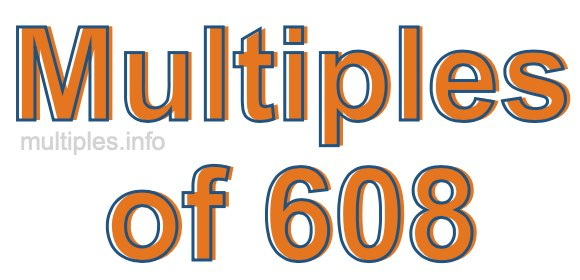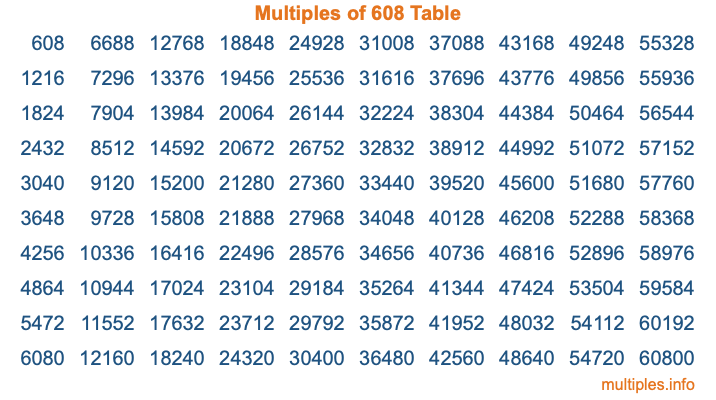Multiples of 608Welcome to the Multiples of 608 page. Here we will first teach you everything you will ever need to know about the multiples of 608, and then give you a study guide summary of everything we taught you to make sure you remember it all. Use this page to look up facts and learn information about the multiples of 608. This page will make you a multiples of six hundred eight expert!

Definition of Multiples of 608
Multiples of 608 are all the numbers that when divided by 608 equal an integer. Each of the multiples of 608 are called a multiple. A multiple of 608 is created by multiplying 608 by an integer.

Therefore, to create a list of multiples of 608, you start with 1 multiplied by 608, then 2 multiplied by 608, then 3 multiplied by 608, and so on for as long as you want. Thus, the list of the first five multiples of 608 is 608, 1216, 1824, 2432, and 3040. To see a larger list of multiples of 608, see the printable image of Multiples of 608 further down on this page. We also have a category where you can choose any nth multiple of 608.

Multiples of 608 Checker
The Multiples of 608 Checker below checks to see if any number of your choice is a multiple of 608. In other words, it checks to see if there is any number (integer) that when multiplied by 608 will equal your number. To do that, we divide your number by 608. If the the quotient is an integer, then your number is a multiple of 608.

Is  a multiple of 608?

Least Common Multiple of 608 and ...
A Least Common Multiple (LCM) is the lowest multiple that two or more numbers have in common. This is also called the smallest common multiple or lowest common multiple and is useful to know when you are adding our subtracting fractions. Enter one or more numbers below (608 is already entered) to find the LCM.

Check out our LCM Calculator if you need more details about the Least Common Multiple or if you need the LCM for different numbers for adding and subtraction fractions.

nth Multiple of 608
As we stated above, 608 is the first multiple of 608, 1216 is the second multiple of 608, 1824 is the third multiple of 608, and so on. Enter a number below to find the nth multiple of 608.

th multiple of 608

Multiples of 608 vs Factors of 608
608 is a multiple of 608 and a factor of 608, but that is where the similarities end. All postive multiples of 608 are 608 or greater than 608. All positive factors of 608 are 608 or less than 608.

Below is the beginning list of multiples of 608 and the factors of 608 so you can compare:

Multiples of 608: 608, 1216, 1824, 2432, 3040, etc.

Factors of 608: 1, 2, 4, 8, 16, 19, 32, 38, 76, 152, 304, 608

As you can see, the multiples of 608 are all the numbers that you can divide by 608 to get a whole number. The factors of 608, on the other hand, are all the whole numbers that you can multiply by another whole number to get 608.

It's also interesting to note that if a number (x) is a factor of 608, then 608 will also be a multiple of that number (x).

Multiples of 608 vs Divisors of 608
The divisors of 608 are all the integers that 608 can be divided by evenly. Below is a list of the divisors of 608.

Divisors of 608: 1, 2, 4, 8, 16, 19, 32, 38, 76, 152, 304, 608

The interesting thing to note here is that if you take any multiple of 608 and divide it by a divisor of 608, you will see that the quotient is an integer.

Multiples of 608 Table
Below is an image of the first 100 multiples of 608 in a table. The table is in chronological order, column by column. The first column has the first ten multiples of 608, the second column has the next ten multiples of 608, and so on.The Multiples of 608 Table is also referred to as the 608 Times Table or Times Table of 608. You are welcome to print out our table for your studies.

Negative Multiples of 608
Although not often discussed or needed in math, it is worth mentioning that you can make a list of negative multiples of 608 by multiplying 608 by -1, then by -2, then by -3, and so on, to get the following list of negative multiples of 608:

-608, -1216, -1824, -2432, -3040, etc.

Multiples of 608 Summary
Below is a summary of important Multiples of 608 facts that we have discussed on this page. To retain the knowledge on this page, we recommend that you read through the summary and explain to yourself or a study partner why they hold true.

There are an infinite number of multiples of 608.

A multiple of 608 divided by 608 will equal a whole number.

608 divided by a factor of 608 equals a divisor of 608.

The nth multiple of 608 is n times 608.

The largest factor of 608 is equal to the first positive multiple of 608.

608 is a multiple of every factor of 608.

608 is a multiple of 608.

A multiple of 608 divided by a divisor of 608 equals an integer.

608 divided by a divisor of 608 equals a factor of 608.

Any integer times 608 will equal a multiple of 608.

Multiples of a Number
Here you can get the multiples of another number, all with the same attention to detail as we did for multiples of 608 on this page.

Multiples of
Multiples of 609
Did you find our page about multiples of six hundred eight educational? Do you want more knowledge? Check out the multiples of the next number on our list!